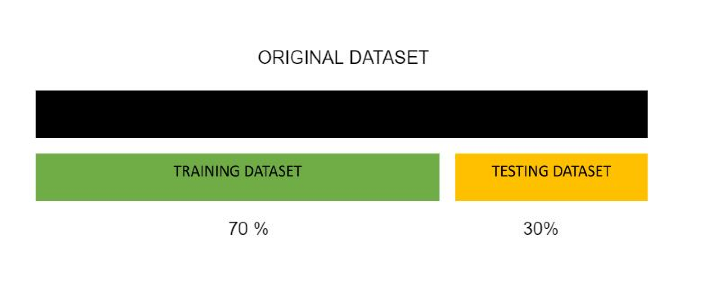# Difference Between Training and Testing Data

## Introduction

In Machine Learning, a good model is generated if we have a good representation and amount of data. Data may be divided into different sets that serve a different purposes while training a model. Two very useful and common sets of data are the training and testing set. The training set is the part of the original dataset used to train the model and find a good fit. Testing data is part of the original data used to validate the model train and analyze the metrics calculated.

In this article lets us explore training and testing data sets in detail.

The Training Data The Testing Data
The data which is provided to the Machine Learning model so that it can learn the patterns in it by analyzing it is called the training dataset. The data that is used to evaluate the model and check its performance, is known as the testing data.
It is generally larger than any of the other sets created. The size of the training dataset is more than the testing dataset. Common ratios that are taken are 80:20,70:30, etc between train and test sets It is generally taken in ratios like 80:20 or 70:30, the smaller ratio representing the testing da
The larger the size of the data, the more information available to the model. However, it should be kept in mind not to train the model the whole data, which might lead to overfitting The data used in testing should be completely unseen so that the model gets new data to check the performance
The data used for training can either have labels or may be unlabelled. This depends upon the type of task. If it is an unsupervised algorithm like KMeans labels are not needed, but if it is a classification task like finding whether an email is spam or not then, labeled trained data is needed. The testing data can be labeled or unlabelled based on the type of task.
The data should be relevant to the use case or problem being solved. For example, to determine the house price, then data related to the location of the house, area, etc are relevant for training The data should represent the original data as in the training dataset and should not completely deviate in characteristics
The training data should be larger so that the model is well-fitted and does not lead to underfitting due to data insufficiency. The dataset should be quite large enough so that the algorithm/model can make better predictions and show some relevant results on the metrics.
For example, let us suppose a Forecasting model that can forecast the sales of a company in the future. For this model to be built and learned it needs historical data from the past. This is provided as training data. For the same forecasting model, a part of the historical data can be used for testing.### Code Implementation

Let us see the implementation of splitting a dataset into training and testing sets and analyze some of its characteristics.

Dataset CSV
import pandas as pd
from sklearn.model_selection import train_test_split

X_data = data.iloc[:, :-1]
y_data = data.iloc[:, -1]

X_train, X_test, y_train, y_test = train_test_split(
X_data, y_data, test_size=0.3)

print("X Train shape : ",X_train.shape)
print("y Train shape : ",y_train.shape)
print("X Test shape : ",X_test.shape)
print("y Test shape : ",y_train.shape)


### Output

X Train shape :  (289, 7)
y Train shape :  (289,)
X Test shape :  (125, 7)
y Test shape :  (289,)


From the above implementation, we have split the original dataset into train and test sets in the ratio of 70:30. The dataset has 7 feature columns in general. Scikit learn’s train_test_split function is a handy and useful tool to split any dataset into train and test splits.

## Conclusion

Both the training set and testing set are subparts of the original data. The training set is used to train the model and testing data is used by the trained model to predict and evaluate the performance of the model on unseen data. The training set is usually larger than the testing set but both datasets should be relevant and obtained from the same source and have similar characteristics

Updated on: 22-Sep-2023

870 Views